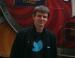# C++ compiler upgrades on infoarenaPaul-Dan Baltescu
27 aprilie 2013

We now have the --std=c++0x compiler option enabled on infoarena. We also updated our g++ compiler to 4.8.

## What does it mean?

C++ users can now use a bunch of cool features, some of which are briefly described below. Keep in mind that these features are not yet available at OJI, ONI, etc., so don't use them at any of these competitions unless they are allowed explicitly by the regulations.

### 1. auto

You can now let the compiler infer the type of your variables with auto:

``````auto a = 45;
auto b = 4.5;
auto c = vector<int>(10);``````

auto can also be used with const auto or const auto&. In most cases, auto cannot be used in function signatures.

### 2. range-based for loops

In C++11, you can write less code to iterate over every element in a list of elements:

``````int array = {1, 2, 3, 4, 5};
for (int x: array) {
cout << x << endl;
}``````

If you want to modify the elements in the list, you need to get a reference to the current element:

``````double array = {1.5, 2.7, 3.9};
for (auto &x: array) {
x *= 2;
}``````

Note: This code compiles without using &, but the original array is not modified unless a reference is used.

### 3. initializer lists

Simple one-line initializations with lists of constant values:

``vector<pair<int, int>> dirs = {{-1, 0}, {0, -1}, {1, 0}, {0, 1}};``

Note that in C++11 you no longer need to introduce a space between closing right angle brackets (>>).

### 4. unordered_set, unordered_map

These are hash-based implementations of the well known set and map containers; the average time complexity on most operations is O(1). (These containers were previously available on infoarena under tr1, but only a small fraction of users were actually using them.)

``````#include <unordered_map>
...
using namespace std;
...
unordered_map<string, int> age = {
{"john", 18}, {"mary", 21}, {"anna", 19}
};
cout << "Anna is " << age["anna"] << " years old." << endl;``````

Using STL vectors, pairs or user defined objects as keys is a little trickier because you also need to provide a hash function.

``````#include <unordered_set>
#include <vector>

using namespace std;

// Some user defined magic constants used for hashing.
const int P = 666013;

struct myhash {
size_t operator()(const vector<int>& v) const {
size_t value = 1;
for (auto x: v) {
value = value * P + hash<int>()(x);
}
return value;
}
};
...
unordered_set<vector<int>, myhash> s;
vector<int> v = {1, 2, 3};
s.insert(v);``````

You don't need to worry about collisions, unordered_set and unordered_map will take care of them for you.

### 5. lambda functions

You can now define anonymous functions to write less code. A common application is sorting according to multiple criteria.

``````#include <algorithm>

using namespace std;

...
vector<int> a = {5, 3, 1, 3};
vector<int> b = {6, 1, 7, 2};
vector<int> ind = {0, 1, 2, 3};

sort(ind.begin(), ind.end(), [&a, &b](int x, int y) -> bool {
return a[x] < a[y] || (a[x] == a[y] && b[x] < b[y]);
});

for (int i: ind) {
cout << a[i] << " " << b[i] << endl;
}``````

(Often, a cleaner alternative to using lambda functions for sorting is to define a class holding all the data for each entry and to implement the < operator.)

Let us know in the comments section what are the C++11 features that you use for programming contests. Code snippets illustrating your favorite tricks are welcome.

Categorii: Features
remote content